# Helical Geometrodynamics - the key -

### Researching one step away from the discovery of the fundamental key. Self-assembling matter in helical geometryThe Romanian Academy publishes, in 2017, the first official paper on the fundamental construction principle of the Universe, a geometric key. This paper is connected to constructal theory, as a logical succession to finding mechanisms for self-assembling matter.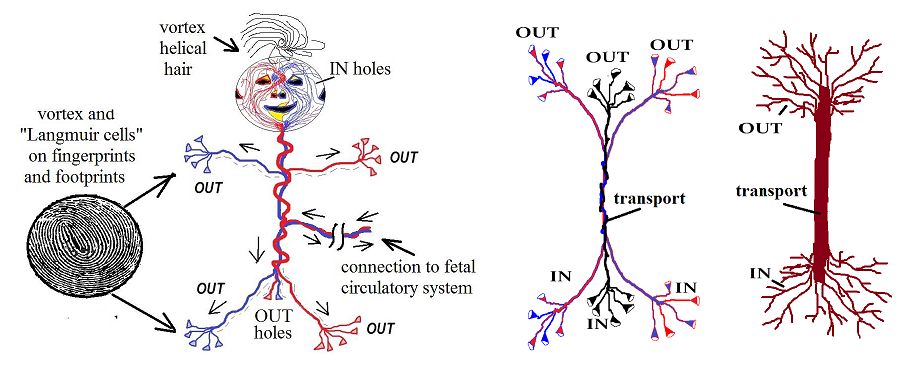# Theory of the Origin, Evolution, and Nature of Life

### Erik D. Andrulis, Department of Molecular Biology and Microbiology, Case Western Reserve University School of Medicine,

This theory is based upon a straightforward and non-mathematical core model and proposes unique yet empirically consistent explanations for major phenomena including, but not limited to, quantum gravity, phase transitions of water, why living systems are predominantly CHNOPS (carbon, hydrogen, nitrogen, oxygen, phosphorus, and sulfur), homochirality of sugars and amino acids, homeoviscous adaptation, triplet code, and DNA mutations. The theoretical framework unifies the macrocosmic and microcosmic realms, validates predicted laws of nature, and solves the puzzle of the origin and evolution of cellular life in the universe. Basic theoretical concepts are built on a chiral model called gyromodel. This gyromodel-supplemented with symbolic variables-is an exemplar for understanding IEM emergence, adaptation, movement, and evolution in the natural world.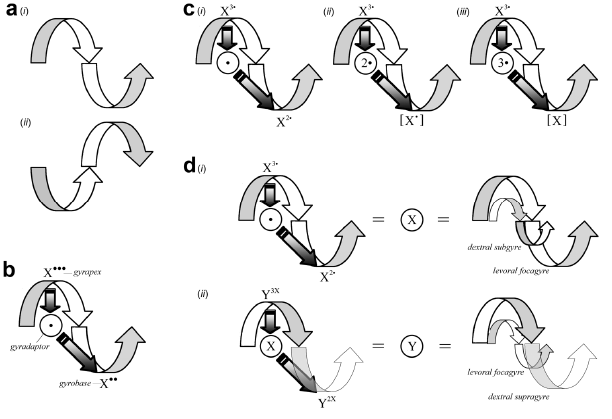https://www.mdpi.com/2075-1729/2/1/1/pdf

The two parts of our brain are adapted for inside systems feedback or outside, for inside work and for outside work. We understand right now that... the molecular chirality make the difference. Our inner world and our outer world is being analyzed distinctly from the two areas in our brain.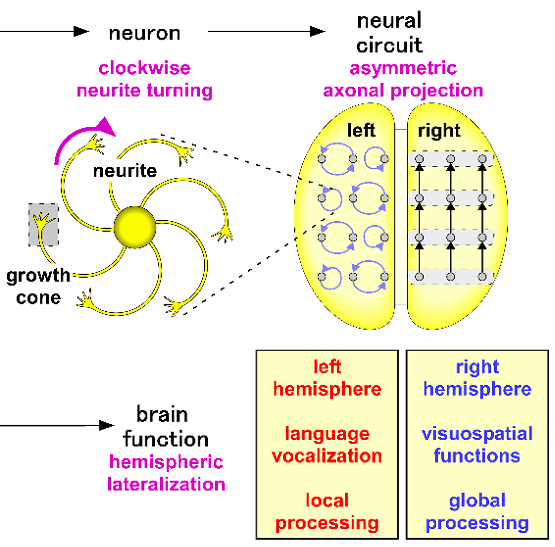Gravitomagnetic equations result from applying quaternionic differential operators to the energy-momentum tensor. These equations are similar to the Maxwell's EM equations. The gravitomagnetic equations turn out to be parent equations generating the following set of equations: (a) the vorticity equation giving solutions of vortices with nonzero vortex cores and with in nite lifetime; (b) the Hamilton-Jacobi equation loaded by the quantum potential. This equation in pair with the continuity equation leads to getting the Schrodinger equation
describing a state of the superfuid quantum medium (a modern version of the old ether); (c) gravitomagnetic wave equations loaded by forces acting on the outer space. These waves obey to the Planck's law of radiation.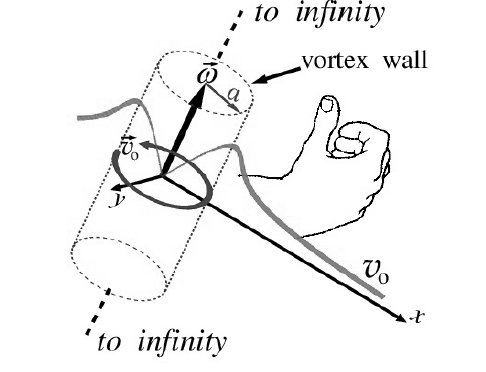Institutes for Theoretical Physics

Fluid Black Holes - gravity as a fluid

Turbulent Black Holes - fluid-gravity corespondence

Research Institutes

Nano-size Superfluidity - nano-size matter as a fluid

Quandum Tornadoes - laser interaction and quantum rotations

Spin filter - Induced Spin Selectivity in biological chiral molecules

Law of Creation and Grand Unifcation Theory - Law of Tao Yin-Yang Creation could be the fundamental principle.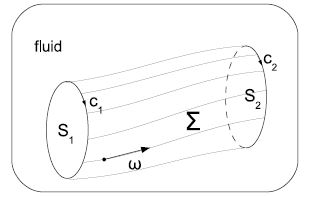"Helmholtz theorem says that, under the flow of ideal barotropic fluid, vortex lines
always move to vortex lines again (so vortex lines are "frozen" into the fluid)...Recall that angular momentum conservation is also an eminence grise behind the "purely geometrical" Kepler's
second law of planetary motion (the same area is swept out in a given time)."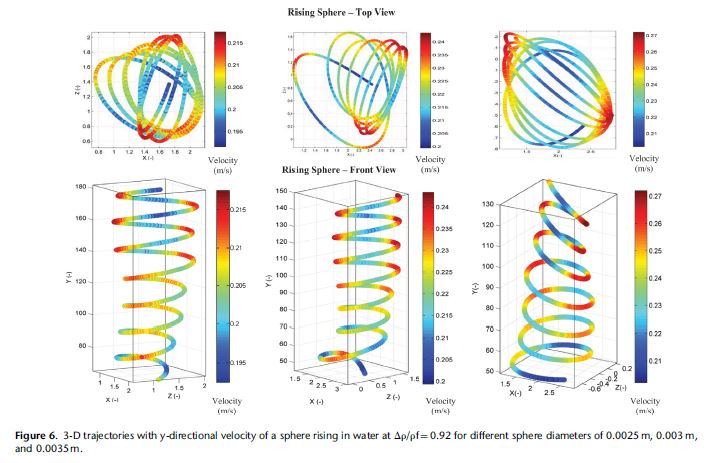"Heavy particles sink straight in water, while buoyant bubbles and spheres may zigzag or spiral as they rise. The precise conditions that trigger such path-instabilities are still not completely understood."  (Really?)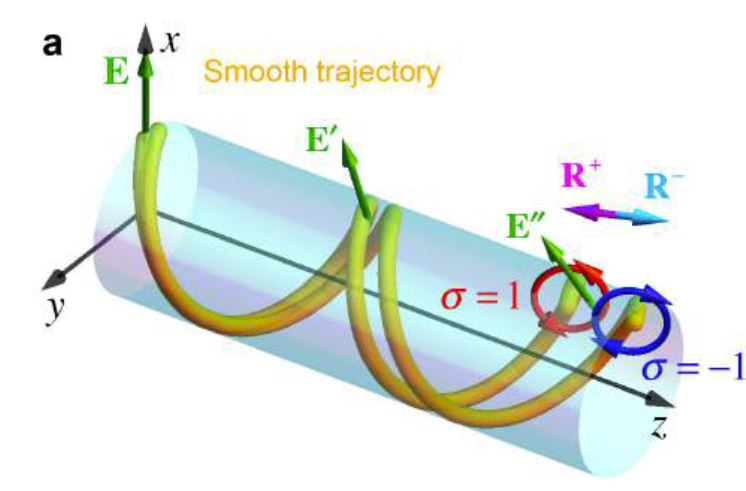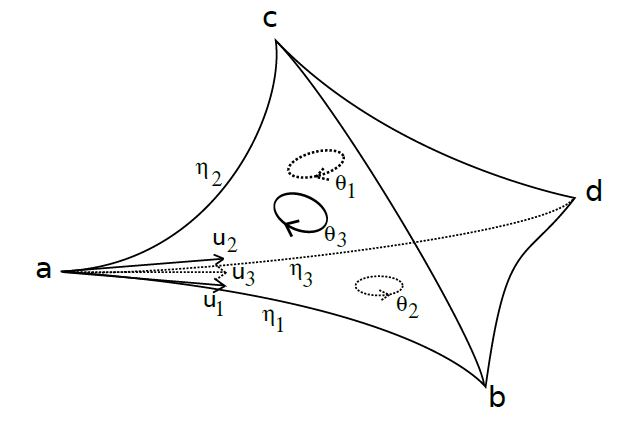"Light carries spin and orbital angular momentum. These dynamical properties are determined by the polarization and spatial degrees of freedom of light.....We show that spin-orbit interactions are inherent in all basic optical processes, and they play a crucial role at subwavelength scales and structures in modern optics."

We investigate the generalization of loop gravity's twisted geometries to a q-deformed gauge group. In the standard undeformmed case, loop gravity is a formulation of general relativity as a diffeomorphism-invariant SU(2) gauge theory... One then glues them allowing for both curvature and torsion... In particular, we introduce two new closure constraints for hyperbolic tetrahedron. One is compact and expressed in terms of normal rotations (group elements in SU(2) associated to the triangles) and the second is non-compact and expressed in terms of triangular matrices (group elements in SB(2,C)).